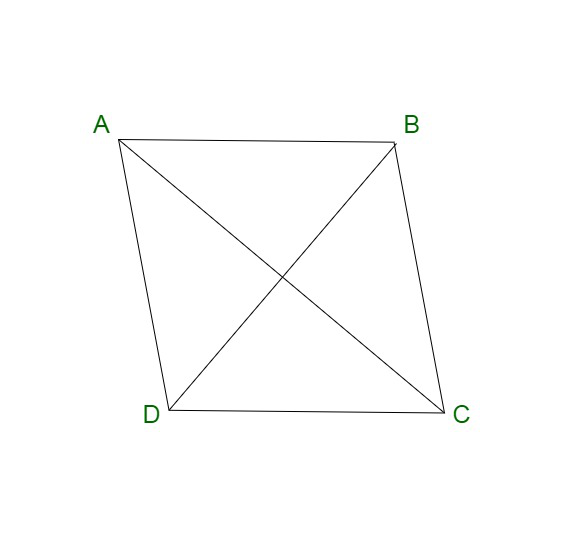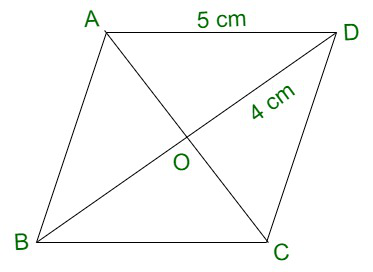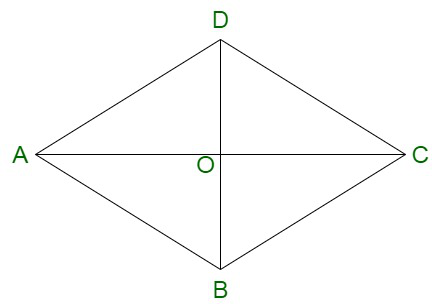GeeksforGeeks App
Open AppBrowser
Continue

# If the length of one side of a rhombus is 5cm and its diagonal is 8cm, find the length of the other diagonal.

Rhombus is also known as a four-sided quadrilateral. It is considered to be a special case of a parallelogram. A rhombus contains parallel opposite sides and equal opposite angles. A rhombus is also known by the name diamond or rhombus diamond. A rhombus contains all the sides of a rhombus as equal in length. Also, the diagonals of a rhombus bisect each other at right angles.Properties of a Rhombus

A rhombus contains the following properties :

• A rhombus contains all equal sides.
• Diagonals of a rhombus bisect each other at right angles.
• The opposite sides of a rhombus are parallel in nature.
• The sum of two adjacent angles of a rhombus is equal to 180o.
• There is no inscribing circle within a rhombus.
• There is no circumscribing circle around a rhombus.
• The diagonals of a rhombus lead to the formation of four right-angled triangles.
• These triangles are congruent to each other.
• Opposite angles of a rhombus are equal.
• When you connect the midpoint of the sides of a rhombus, a rectangle is formed.
• When the midpoints of half the diagonal are connected, another rhombus is formed.

### Diagonal of a Rhombus

A rhombus has four edges joined by vertices. On connecting the opposite vertices of a rhombus, additional edges are formed, which result in the formation of diagonals of a rhombus. Therefore, a rhombus can have two diagonals each of which intersects at an angle of 90°.

Properties of diagonal of a rhombus

The diagonals of a rhombus have the following properties:

• The diagonals bisect each other at right angles.
• The diagonals of a rhombus divide into four congruent right-angled triangles.
• The diagonals of a rhombus may or may not be equal in length.

Computation of diagonal of rhombus

The length of the diagonals of the rhombus can be calculated by using the following methods:

By Pythagoras Theorem

Let us assume d1 to be the diagonal of the rhombus.

Since, we know, all adjacent sides in a rhombus subtend an angle of 90 degrees between them.

Therefore if we consider the rhombus ABCD,

In the triangle, BCD we have,

Since,

Perpendicular2 + Base2 = Hypotenuse2

Putting the line segments,

BC2 + CD2 = BD2

Now, we have,

In the case of a square rhombus, with all sides equal,

We know,

Square Diagonal: a√2

where a is the length of the side of the square

In case of a rectangle rhombus, we have,

Rectangle Diagonal: √[l2 + b2]

where,

• l is the length of the rectangle.
• b is the breadth of the rectangle.

### If the length of one side of a rhombus is 5cm and its diagonal is 8cm, find the length of the other diagonal.

Solution:Since, we have,

Length of diagonal of the rhombus = 4 cm

Length of the side of the rhombus = 5 cm

We know, Diagonals of a rhombus bisect each other and they are perpendicular to each other.

Let us consider half of the length of second diagonal to be x.

Since, both the diagonals and a side form right angled triangle in case of a rhombus.

Therefore,

By Pythagoras theorem, we have,

Perpendicular 2 + Base 2 = Hypotenuse 2

⇒ x2 + 42 = 52

⇒ x2 = 25 – 16

⇒ x2 = 9

⇒ x = √9

⇒ x = 3

Thus, we get, length of second Diagonal d2 = 2x

⇒ 2 × 3 = 6 cm

Therefore,

The length of second diagonal d2 is 6 cm.

### Sample Questions

Question 1. The area of a rhombus is 10 cm sq. If the length of a diagonal is twice as long as the other diagonal. Then find the length of both the diagonals?

Solution:

Assume,

The shorter diagonal be d1

The longer diagonal be d2

As it is given in the problem that the longer diagonal is twice the shorter diagonal

Thus this can be written as

d2 = 2 × d1……….equation (1)

The area of the Rhombus is

A =10 =10 =……..from equation (1)

10 =d1 = √10

d2 = 2 × √10

d2 = 2√10

Question 2. Calculate the area of the rhombus that has each side 34 cm and the measure of its one diagonals is 32 cm.

Solution:Here assume ABCD is a rhombus

Thus,

34 cm = AB = BC = CD = AD

Diagonal BD = 32 cm

Here O is the diagonal intersection point

Thus,

BO = OD = 16 cm

Further.

In the ∆ AOD,

⇒ 342 = AO2 + 162

⇒ 1156 = AO2 + 256

⇒ AO2 = 1156 – 256

⇒ AO2 = 900

⇒ AO = 30 cm

As,

AC = 2 × AO

= 2 × 30

AC = 60 cm

Area of rhombus == 960 cm2

Question 3. Calculate the altitude of the rhombus having an area of 630 cm² and its perimeter is 360 cm.

Solution:

Here it is given that

The perimeter of rhombus is 360 cm

Therefore,

The side of rhombus === 90 cm

Now,

The area of rhombus = base × height

⇒ 630 = 90 × h

⇒ h =⇒ h =7 cm

Hence,

The altitude of the rhombus is 7 cm.

Question 4. A hall of a building consists of 4000 rhombus-shaped tiles having diagonals 80 cm and 50 cm. Calculate the cost of buffing of all the tiles of the hall at the cost of ₹20 per square meter?

Solution:

Here it is given that the length of the diagonals of each rhombus-shaped tile is 80 cm and 50 cm.

Thus,

The area of one tiles is; a =a =a = 2000 sq. cm

Changing the area is square metre

a =a = 0.2 sq. metre

Further the area for 4000 tiles will be; = 4000 × 0.2 sq.m

= 800 sq.m

Cost of buffing 1 sq.m is ₹ 20

Cost of buffing 800 sq.m will be; 800 × 20

= ₹1600

Question 5. Assume the diagonals of a rhombus-shaped glass are 15 cm and 24 cm. Calculate the area of the Rhombus glass?

Solution:

We know,

Area of rhombus =a =a = 180 sq.cm

Therefore,

The area of the Rhombus shapes glass is 180 sq.cm.

My Personal Notes arrow_drop_up
Related Tutorials